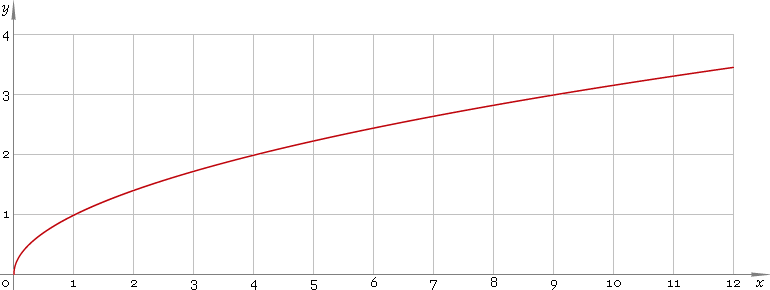The Art of Interface

# √ or sqrt — square root function

Category. Mathematics.

Abstract. Square root: definition, graph, properties and identities.

## 1. Definition

Square root function is inverse of the power function with power a = 2

x2

The square root is denoted with radical symbol:

x

Square root is equivalent to the power of one second:

xx1/2

## 2. Graph

Square root function defined for non-negative part of real axis — so, its domain is [0, +∞). Function graph is depicted below — fig. 1.Fig. 1. Graph of the square root function y = √x.

Function codomain non-negative part of the real axis: [0, +∞).

## 3. Identities

Take into account, that because of square root defined only for non-negative values, and power of two defined everywhere, the order of these two functions makes difference:

x2x
√(x2) ≡ |x|

and as well

x ≡ signx √(x2)

Reciprocal argument:

√(1/x) = 1 /√x

Product and ratio of arguments:

√(xy) = √|x|√|y|
√(x/y) = √|x| /√|y|

Power of argument:

√(xa) = √|x|a ≡ |x|a/2

## 4. Solution of quadratic equation

ax2 + bx + c = 0

has roots

x = [−b ± √(b2 − 4ac)] /(2a)

For equation with even coefficient for the first power ax2 + 2bx + c = 0

roots have simplified form

x = [−b ± √(b2ac)] /a

## 5. Solution of normalized quadratic equation

Normalized quadratic equation x2 + bx + c = 0

has roots

x = [−b ± √(b2 − 4c)] /2

And equation with even coefficient for the first power x2 + 2bx + c = 0

has the simplest form for its roots

x = −b ± √(b2c)

## 6. Support

Square root function or sqrt is supported in:

Square root function of the complex argument or sqrt is supported in:

## 7. How to use

To calculate square root of the number:

``sqrt(2);``

or

``√(2);``

To calculate square root of the current result:

``sqrt(Rslt);``

or

``√(Rslt);``

To calculate square root of the number x in memory:

``sqrt(Mem[x]);``

or

``√(Mem[x]);``